# Circular References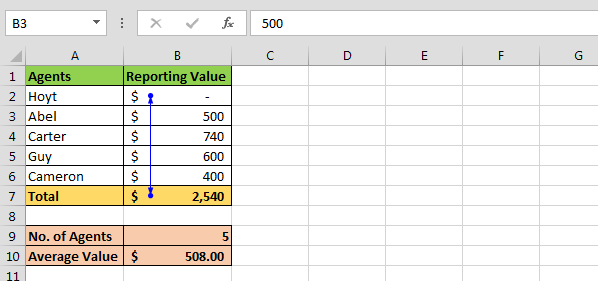Circular reference can be a formula that refers to its own cell value, or refers to a cell dependent on its own cell value.

In simple words, the cell in which formula is being calculated & the cell itself is also included or part of the range then a circular reference warning message will appear. It might cause the incorrect calculation by the formulas.

Let us take an example:

• In cell A1 & A2, numbers are entered & in cell A3 we want to find out the total.
•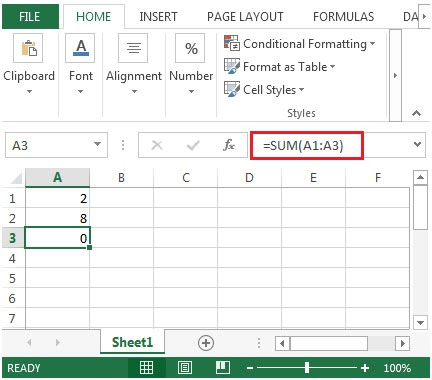While typing the sum formula, the range is stretched to cell A3 in which formula is entered.
• Excel will pop-up an error message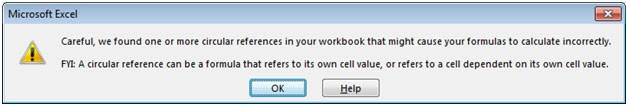If above shown error message regarding circular reference pops up while adding formulas, then you might have created circular cell reference unknowingly. You can track & then remove the incorrect reference & it will remove the circular reference.

To track circular reference, follow the below steps:

• Click on blank cell
• Click on Formulas ribbon
• In Formula Auditing group, click on Error Checking
• Select Circular References & it will provide the location of the cell in which circular reference occurs.
•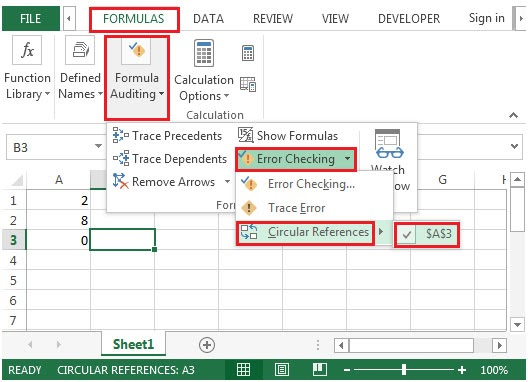• If there are multiple circular references, then you are required to manually go to each cell & correct the formula until the status bar no longer shows the word “CIRCULAR REFERENCES”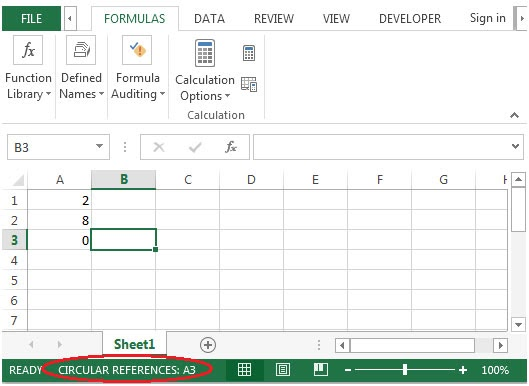If we use Excel for any length of time to create the dashboards, templates, reporting format, etc. in which we have to use formulas then the possibility is to create a formula that results in a circular reference. In this article, you will learn about Circular reference in Microsoft Excel. What is the use of Circular reference?

Let’s take another example and understand:-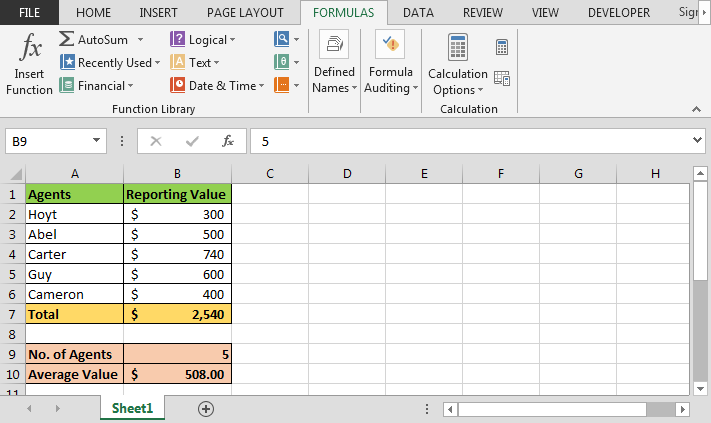In the above-shown image, we have agent data. Below the data, we have a summary for a number of agents and average scored value. To calculate the number of agents we have used COUNTA function and for average we have to use the Average function.

• Enter the formula in cell B2
• =B7-SUM(B3:B6)
•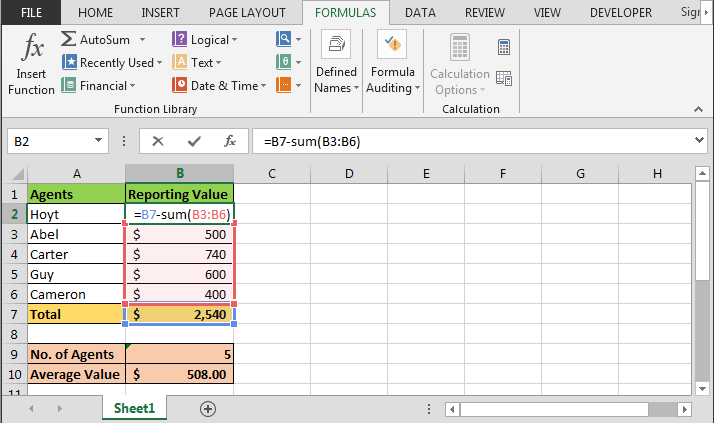• Press Enter on your keyboard
•Now to check the reason of showing the circular reference follow the steps below:-

• Go to Formulas Tab.
• In the group of Formula Auditing
• Click on Error Checking
• Click on Circular Reference
•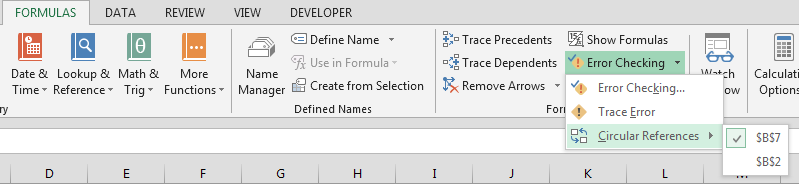• In the drop down list you can find the cell references

Conclusion: - When you are working in Excel sheet for templates and dashboard this problem is very common so it occurs. This article will be helpful to rectify this kind of problem while working in Excel.

### How to allow Circular Referencing in Excel?

On the Excel ribbon, click on the File button. Go to Options.  Excel Options Dialog will open.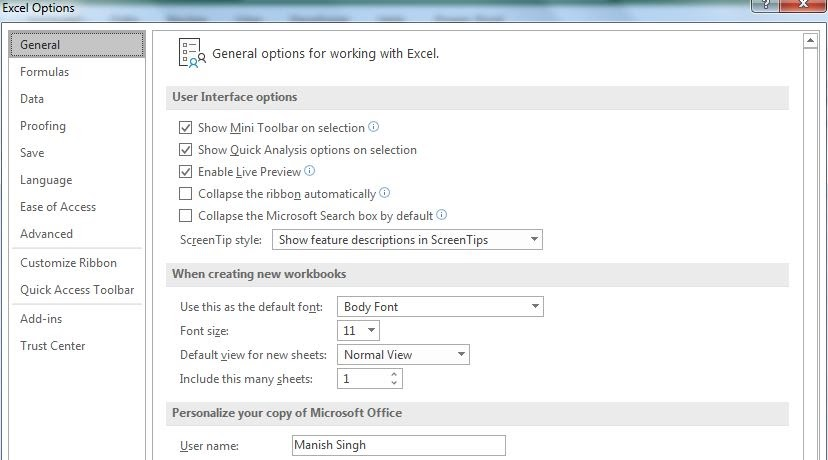Click on the Formula option. Here you will find Allow iterative calculations. Check to allow circular calculations. You can control the number of iterations and maximum change too.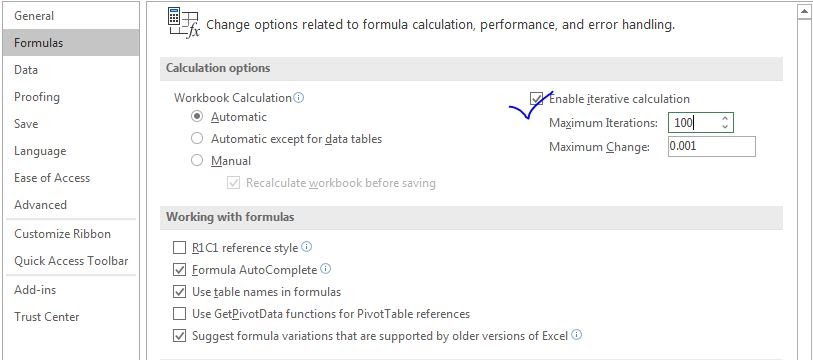This will allow the circular referencing, and excel will not show any error when do circular referencing but you might not get the answer you wanted.

Some of the observational notes using the circular reference in Excel.

Notes :

1. The formula only works with numbers.
2. This tool helps in keeping track of the references cell and referencing cell both.

Below you can find more examples:-

How we can see circular reference

If you liked our blogs, share it with your friends on Facebook. And also you can follow us on Twitter and Facebook.We would love to hear from you, do let us know how we can improve, complement or innovate our work and make it better for you. Write us at info@exceltip.com

Related Articles:

What is an Absolute Reference in Excel | In many of my articles, you must have read the word absolute reference. If not, you must have noticed the \$ (dollar) sign before cell references (\$A\$1). They are absolute references. When we prefix the \$ sign before

Expanding References in Excel | The ranges that expand when copied in the below cells or to right cells are called expanding references. Where do we need expanding references? Well, there were many scenarios

Relative and Absolute Reference in Excel | Understanding of Relative and Absolute Reference in Excel is very important to work effectively on Excel. Relative and Absolute referencing of cells and ranges

Dynamic Worksheet Reference | Dynamic worksheet reference suggests that using a value in one sheet from another. The INDIRECT function is a cell reference function that allows us to make the text reference into actual reference.

Popular Articles:

50 Excel Shortcuts to Increase Your Productivity | Get faster at your task. These 50 shortcuts will make you work even faster on Excel.

How to use Excel VLOOKUP Function| This is one of the most used and popular functions of excel that is used to lookup value from different ranges and sheets.

How to use the Excel COUNTIF Function| Count values with conditions using this amazing function. You don't need to filter your data to count specific value. Countif function is essential to prepare your dashboard.

How to use the SUMIF Function in Excel | This is another dashboard essential function. This helps you sum up values on specific conditions.

Terms and Conditions of use

The applications/code on this site are distributed as is and without warranties or liability. In no event shall the owner of the copyrights, or the authors of the applications/code be liable for any loss of profit, any problems or any damage resulting from the use or evaluation of the applications/code.